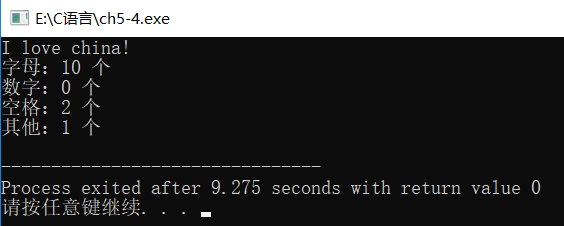# C语言代码如下：

``````#include <stdio.h>

int main() {
char c;
int alpha=0, number=0, space=0, other=0;

c = getchar();//输入一个字符给变量 c
while(c != '\n'){
if((c>='A' && c<='Z') || (c>='a' && c<='z')){//判断是否为字母
alpha = alpha+1;
}else if(c>='0' && c<='9'){//判断是否为数字
number = number+1;
}else if(c == ' '){//判断是否为空格
space = space+1;
}else{//其他
other = other+1;
}
c = getchar();//在输入下一个字符赋值给变量 c
}

printf("字母：%d 个\n", alpha);
printf("数字：%d 个\n", number);
printf("空格：%d 个\n", space);
printf("其他：%d 个\n", other);
}``````

# 运行结果

``````I love china!

--------------------------------
Process exited after 9.275 seconds with return value 0``````